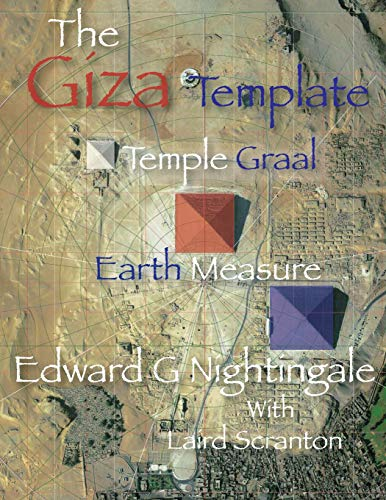Edward Nightingale has published a follow-up article to support his theories which you can read here.

I am submitting a follow-up article that is focused more on the pyramids, their measure and connection to the precessional cycle that may help the reader to better understand the article The Leo, Orion Relationship. The Giza Pyramids and the Precessional Cycle article covers topics that are discussed regularly on GrahamHancock.com and hopefully will generate more discussion. This article will also provide more of a foundation that fully supports the new data I have presented on the precessional cycle encoded at Giza.

The architects, engineers and astronomers that were behind the design of the repository at Giza used a specific template consisting of a circle with a diameter of 9 units and a square of 8 x 8 of the same units. The circle with a diameter of 9 units has the area of 63.6428 when calculated. A square with a side length of 8 of the same size units, has an area of 64. This simple calculation approximates the area of a circle. The 9 unit circle and the 8 x 8 square are used as the survey plat for the design of the Giza repository. It is also a fundamental concept of land management used in many ancient cultures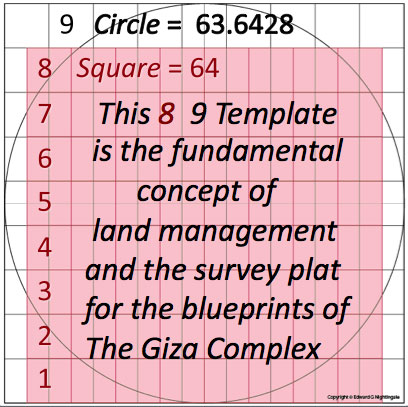Beginning with the 8 x 8 grid the designers applied numbers to the grid to create a coordinate system. The numbers applied to the 8 x 8 square are given to us by Plato in his book Timeaus as the Lambda sequence stating that these were the numbers that God used to create the "Cosmic Soul". Lambda is the 11th letter of the Greek alphabet. The Lambda sequence of numbers given by Plato are, (1,2,4,8 and 1,3,9,27) a doubling sequence (x2) and a tripling sequence (x3). The doubling sequence applied to the X axis (down the left side of the 8 x 8) and a tripling sequence 1,3,9,27) across the top. These numbers continue both down and across to complete the 8 x 8 square.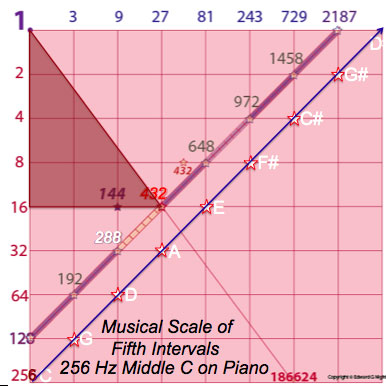There are two diagonal scales shown within the grid, one beginning at 256, this is a Musical scale of perfect fifth intervals. 256 Hertz,(a measure of frequency) is a middle C note on a piano.

The second scale beginning at 128 is where the designers obtained all the units of measure, the inch, foot, and six different cubit lengths. These cubits measurements are, the Light Cubit, the Root Cubit, Royal Cubit, Canonical, Geographic and the Sacred Cubits. These units are all related to each other by specific fractions.

Beginning at 128 on the scale, the number 432 is three intervals up the diagonal scale of 7 intervals (7 down the X axis, 7 across the Y axis and 7 up the diagonal). Each interval of the 7 are then divided by 7 creating a total number of intervals as 49 (7 x 7) on the diagonal scale. 432 is 21st interval up the scale (3 x 7).

The numbers of 21 and 432 are the key to determining the dimensions of all the pyramids. Beginning with 432 and subtracting 21 = 411, this is the base length assigned to the pyramid of Khafre. The slope angle is 53.13 Degrees, a 3,4,5 Pythagorean Triangle. This angle is a is created on the Grid by 4 units down (16) the side and 3 units (27) across the top producing the coordinate of 432, 16 x 27 = 432. This completes the overall dimensions the pyramid of Khafre (G2).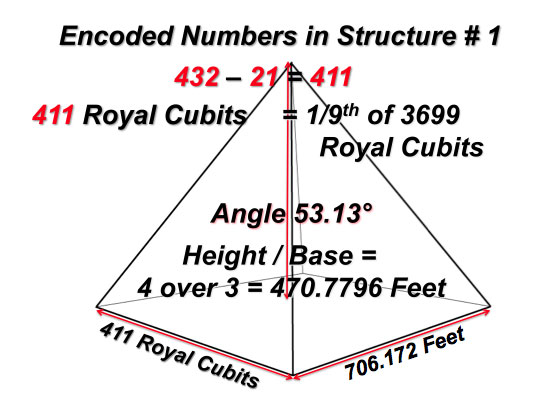To determine the base length of Khufu (G1) the Great Pyramid the number 432 is multiplied by 21 = 9072. The angle of Khufu (G1) is created by the relationship of the circle to the approximate Phi division(13/21 of the Fibonacci sequence) of the square. The division creates a Golden Rectangle in which a Fibonacci Spiral is created. The angle is 51.84 degrees.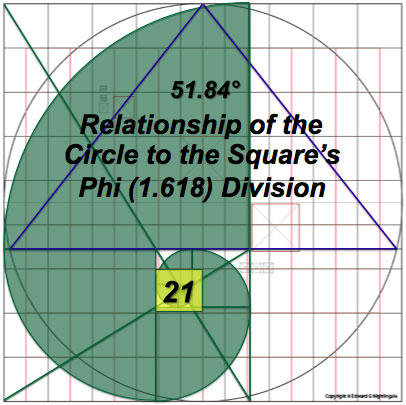The numbers imbedded within this structure, Khufu or G1, a few are shown in this graphic, they include the units of measure, the inch, foot, and six different cubit lengths. The Light Cubit, the Root Cubit, Royal Cubit, Canonical, Geographic and the Sacred Cubits. These units are all related to each other by specific fractions.

These units are all created from this original equation of 432 divided by 21 = 20.571428. This is the KEY number or unit from which all other units of measure are created and is designated the value of Inches. The length of 20.571428 Inches is equal to the length of 1 Root Cubit. From this number, all related cubit dimensions are derived by adding specific fractions to each creating the next cubit length.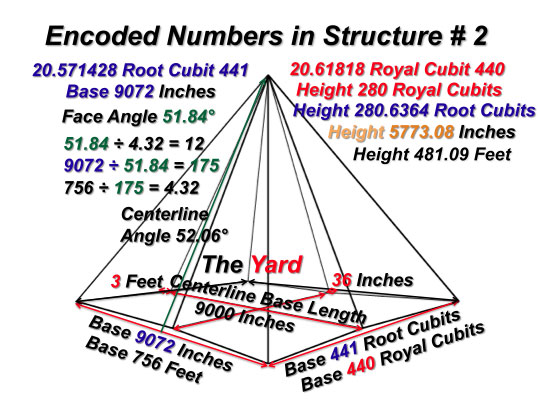For the design of Menkaure (G3), the architects embedded the diagonal scale of 7 down and 7 across and 7 diagonal. There are two different base lengths assigned to this pyramid. One by this equation, 7 x 7 x 7 = 343 (feet), this 343 feet can be seen as 49 units of 7 feet (49 the number of intervals in the diagonal scale) 49 x 7 = 343. The second base length is created by using 48 of these units of 7 feet creating a base length of 336 feet. 48 x 7 = 336 feet. The height of Menkaure (G3) is assigned 4/9ths the height of Khufu (G1) equaling 213.8177 feet. This creates two separate slope angles from the two base lengths, 51.84 degrees and 51.27 degrees.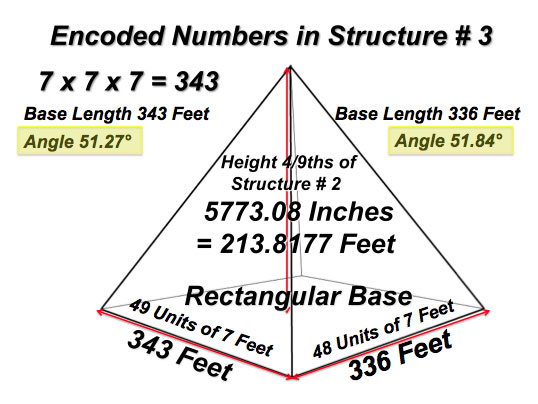The number 51.84 and 51.27 are KEY numbers related to the precessional cycle in that 5184 (years) is 1/5th of the approximate duration of the precessional cycle of 25920 (years) 5 x 5184 = 25920.

The number 5127 is the duration of the calendar used by the Mayans, 2013 (2012 winter solstice completing 2012 years) or the Gregorian date of 12/21/2012. 2013 – 5127 = -3114. That would be the year 3114 BC, the beginning of the Mayan calendar. Two calendars are created here, one beginning with 0 with a duration of 5127 years. A second beginning with -3114 BC and ending with 2013 AD, a duration of 5127 years. A third calendar is created with the number 5773 which is the height in inches of the pyramid of Khufu (G1). This number 5773 corresponds to the 4/9ths height of Menkaure (G3). This number is multiplied by two, 5773 x 2 = 11546, this number is divided by 9 and = 2886.5. This number multiplied by 9 = 25978. 25978 is the approximate duration of the precessional cycle. 5773 is the height of Khufu (G1) in inches, in feet = 481.09 feet. Adding 481.09 to 25978 = 26459.59, in whole numbers, 26460. the full duration of 1 cycle of precession. Confirmation of this is demonstrated in the following equations using the fraction of 49/48ths.

The pyramid of Menkaure (G3) encodes the numbers 49 and 48 in its base lengths as demonstrated. Beginning with 25920 as the (Root) approximate duration of the precessional cycle we are able to correct the approximate duration of the cycle to the observed duration of the cycle by the use of fractions, converting 25920 into the fraction of 48/48ths, 25920 divided by 48 = 540. 25920 + 540 = 26460, the duration of 1 precessional cycle.

What is encoded in the pyramid of Menkaure (G3) is the approximate duration of 1/5 of the 25920 year cycle to the precise duration of the cycle described by Plato as the Great Year. This approximate duration is then adjusted in the manner described to the correct duration of the cycle of 26460 years.

If what I have presented is factual, then it will be easily confirmed or not by what we observe from the view of the Sphinx using star viewing software, in this case Stellarium software.

This first image of the sky is viewed from the Sphinx on the (binary) date of 12/21/2012 0hrs 0min 0sec, the end/beginning of the precessional cycle. This date is by design and is precisely sunrise from the view of the Sphinx. The alignment of the Galactic Plane (in white) and the Ecliptic (in red) create an X and intersect with the Sun at precisely Sunrise. Note: The atmosphere and ground are not shown is these two images for clarity.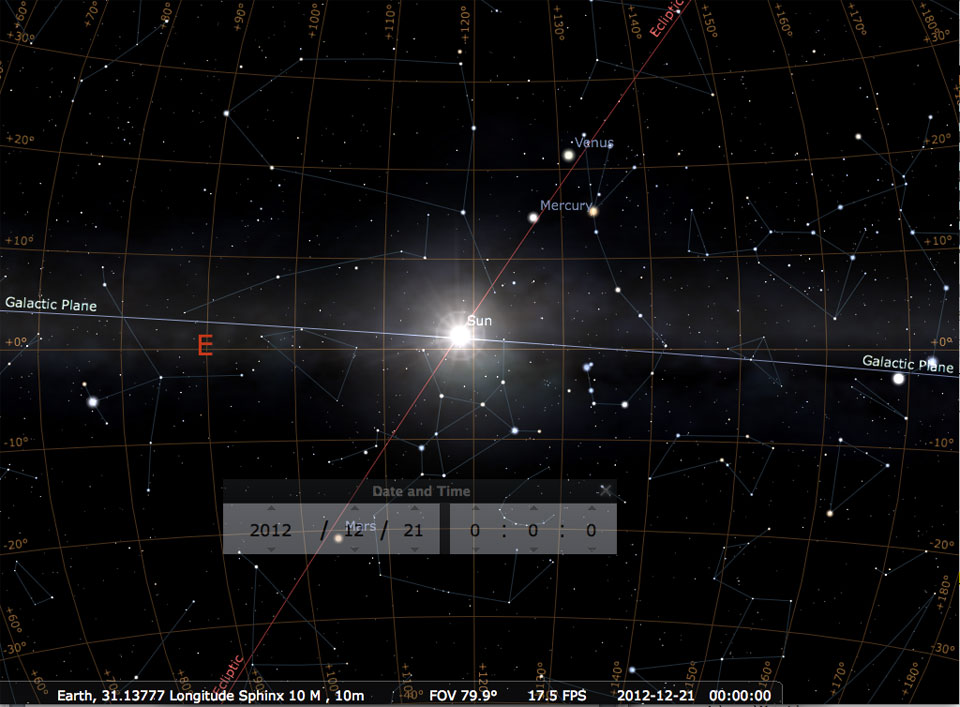The Sun is rising approximately 27 degrees South of East on the horizon.

I have proposed that 12/21/2012 as the end of one cycle and the beginning of another. If I am correct with the calculations gleaned from the design of the three main pyramids, we should witness this same Galactic/ Ecliptic/Sun alignment forming an X with the Sun, 26460 years before 2013. 26460 – 2013 – 24447BC. This image is taken in the year -24447 BC at sunrise. Take notice of the hours and minutes this sunrise occurs. 20hrs and 12minutes after the sunrise recorded in 2012. This alignment only occurs on these two dates with a cycle duration of 26460 years. It is most interesting to note, and no mere coincidence that the angle of the Galactic Plane is 23.5 degrees off 90 degrees, (the pole or axis of the Earth) to the Horizon. 23.5 degrees is the angle of the tilt of Earths axis to the Ecliptic. Also the angle of the ecliptic to the Horizon is 53.13 degrees, the angle of the Pythagorean 3,4,5 Triangle, the slope angle of Khafre (G2).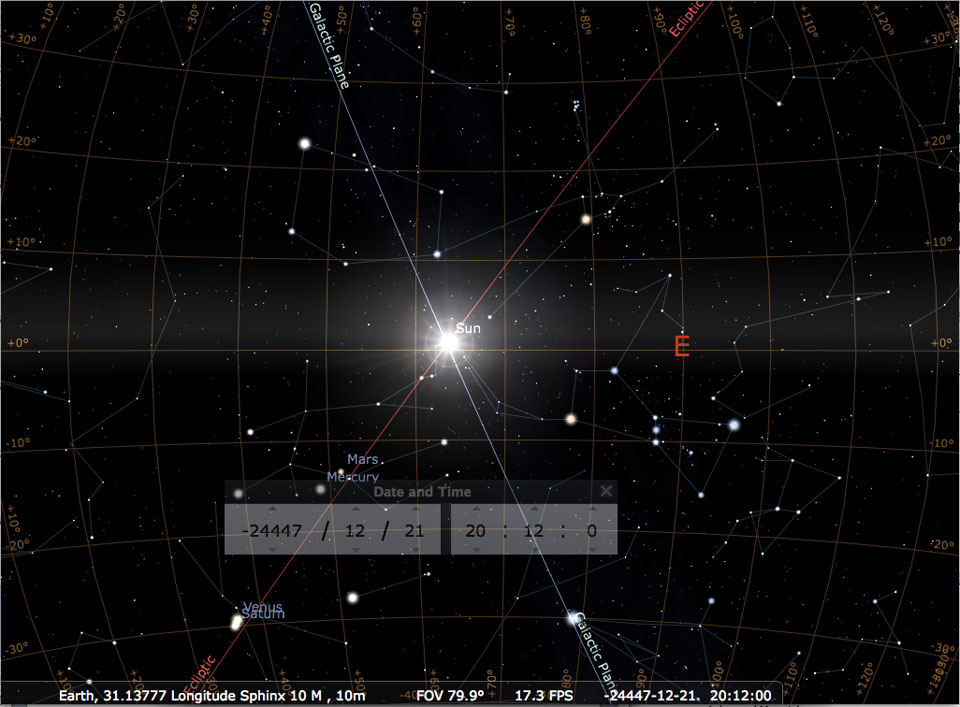The Sun is rising approximately 27 degrees North of East on the horizon, a movement of 54 degrees on the horizon from the end of the cycle to the beginning of the cycle.

In my article The Leo, Orion Relationship [http://www.grahamhancock.com/forum/NightingaleE3.php] I suggested that the designers of Giza encoded the trajectory of our Sun, Sirius and the Pleiades as spiral in nature, originating from the Great Orion Nebula, indexing with the three pyramids as the Orion Correlation Theory states. The Leo aspect of the relationship is the spin view of the Ecliptic.

I have demonstrated a model of the precessional cycle with a beginning and completion. I also propose that certain structures were constructed at precise points and intervals of this cycle. These intervals relate directly to the geological and other evidence of catastrophic events on Earth as documented by Randall Carlson.

The implications and ramifications as a result of the information presented here and in The Giza Template, Temple Graal, Earth Measure are left for the reader to consider.

For more in depth information the book, The Giza Template Temple Graal, Earth Measure is available through Amazon.com at this address:

http://www.amazon.com/exec/obidos/ASIN/1502493233/theofficialgraha

www.thegizatemplate.com

### Biography

Edward Nightingale is an independent researcher, author and speaker who as a Master Woodworker with over 33 years of drawing, measuring and building experience has spent the past 17 years utilizing these skill sets to reverse engineer the Giza Complex.

Edward travelled to Egypt in 1997 in search of evidence for his theory on the architectural design of the Giza pyramids and the Sphinx, the results of his efforts are published in his new book, The Giza Template: Temple Graal, Earth Measure. The book documents the step by step design that the architects of Giza used to encode highly scientific data into geometric and mathematical information to be preserved and passed on to future generations.

Edward’s claims are backed with precise geometry, mathematics, satellite imaging, astronomical charting and cosmology that can be reproduced using the scientific method.

Edward is working on a sequel, Heavens Measure to follow his book Earth Measure.

Website: www.thegizatemplate.com

Edward Nightingale is an independent researcher, author and speaker who as a Master Woodworker with over 33 years of drawing, measuring and building experience has spent the past 17 years utilizing these skill sets to reverse engineer the Giza Complex.

Edward travelled to Egypt in 1997 in search of evidence for his theory on the architectural design of the Giza pyramids and the Sphinx, the results of his efforts are published in his new book, The Giza Template: Temple Graal, Earth Measure. The book documents the step by step design that the architects of Giza used to encode highly scientific data into geometric and mathematical information to be preserved and passed on to future generations.

Edward’s claims are backed with precise geometry, mathematics, satellite imaging, astronomical charting and cosmology that can be reproduced using the scientific method.

Edward is working on a sequel, Heavens Measure to follow his book Earth Measure.

Website: www.thegizatemplate.com

## One thought on “The Giza Pyramids and the Precessional Cycle”

1. Gőczey András says:

Dear Edward, I am a hungarian architect. I made a lot of interesting facts about Giza pyramids. Khufu is an every day calendar for 182 days and back the whole year. Khefren is a half year calendar. Menkaure with their small pyramids a very exact calendar with Moon. I can send you more details about it, the last film of it is -Giza site plan Sun Moon Goczey Andras- I think it is very interestig to know it. best wishews, Andras